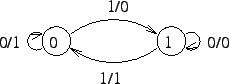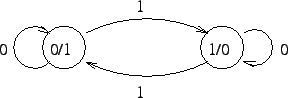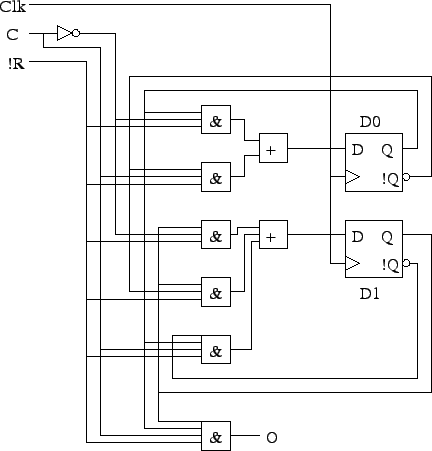Sequential Circuit Analysis

Tom Kelliher, CS 240

Apr. 7, 2010

Announcements

Xilinx assignment due Friday.

Exam on 4/14, covering 4.1-5.2, including material covered in class on addition.

From Last Time

Flip-Flops and waveforms.

Outline

1. Reverse engineering a sequential circuit.

2. Input equations, state tables, and state diagrams.

3. Example problems.

Coming Up

Sequential circuit design.

Reverse Engineering Sequential Circuits

Analysis = reverse engineering.

One doesn't ordinarily do this, but doing so will help with sequential circuit design.

Input Equations, State Tables, and State Diagrams

Consider the following sequential circuit:What does it do -- high level?

What happens if we use !Q as the input to the NEXOR rather than Q?

Mealy (this circuit) vs. Moore machines.

Input Equations

What are the equations for `O` and `D` (input equation)?

Do these differ that much from anything we've already seen?

State Tables

State tables are similar to truth tables, with two additions:

1. Present state inputs -- flip-flop outputs.

2. Next state outputs -- derived from input equations for flip-flops.

 Present State I Next State O 0 0 0 1 0 1 1 0 1 0 1 0 1 1 0 1

State Diagrams

Conveys same information as state table, in a visual form.

State diagram for our example (Mealy):Moore machine example:Example

Determine input and output equations, state table, and state diagram for this circuit:Is this Mealy or Moore?

Thomas P. Kelliher 2010-04-06
Tom Kelliher